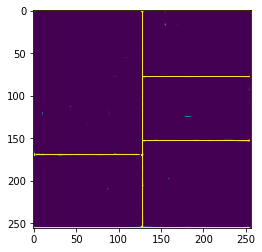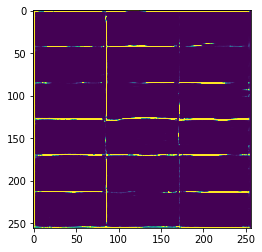Draw Bounding Boxes of Mosaic Picture with Unet
2019-11-14
category: MachineLearning

## Problem

sometimes, the thumbnail picture on DBpedia is a combination of a few pictures, like below:But what I need are separated pictures.

## Solution

Usually, to classify a image, we use CNN layer to filter its features, then Dense layer to classify. Here, I don’t need to classify images, but only to get their features. In another word, I input a matrix representing the original picture, the model ouputs another matrix representing bounding boxes, like below:### 1. Choose Model

I directly used Unet from here:Implementation of deep learning framework – Unet, using Keras

### 2. Collect Data

I used 5 pictures from DBpedia’s thumbnail to train this model. Before using Unet, I did this task with only openCV, so I already have the coordinates of each bounding box.

e.g. this dear picture’s data are:

``````urls = ["https://upload.wikimedia.org/wikipedia/commons/thumb/d/d9/Family_Cervidae_five_species.jpg/300px-Family_Cervidae_five_species.jpg"]
# [startX, startY, endX, endY]
coordinates = [[0,0,225,150],[225,0,338,150],[0,150,104,299],[104,150,203,299],[203,150,338,299]]
``````

### 3. Preprocess Data

firstly, I have to get original picture form url and resize it into proper size(because CNN model’s input size is fixed). the height and width parameter has to be divided by 2, because Unet uses MaxPooling2D and UpSampling2D. Recommended input size in this case is 256 * 256, becase all the thumbnail’s width is 300.

``````def get_image(url, height, width):
response = requests.get(url)
img = Image.open(BytesIO(response.content))
scale = [height/img.size,width/img.size]
img = img.resize((height,width))
imgArray = np.asarray(img)
return imgArray, scale
``````

Secondly, I filter all unnecessary data to help the model to work better. such as blurring it, converting it into a gray picture and rewritting any number bigger than 0 as 1. Then add a dimension for inputing into the model.

``````imgArray = cv2.blur(imgArray, (2,2))
edges = cv2.Canny(imgArray, 0, 250)
edges[edges > 0] = 1
edges = np.asarray(edges, dtype=int)
x = np.expand_dims(edges, axis=2)
``````

Thirdly, resize the coordinates according to the scale I got above. Then draw bounding boxes according to coordinates.

``````y = np.zeros((input_dim,input_dim,1),dtype=int)
for co in coordinate:
co = int(co * scale)
co = int(co * scale)
co = int(co * scale)
co = int(co * scale)
y[co,co:co,0] = 1
y[co,co:co,0] = 1
y[co:co,co,0] = 1
y[co:co,co,0] = 1
``````

Lastly, convert them as numpy arrays

``````train_x = np.asarray(train_x)
train_y = np.asarray(train_y)
``````

### 4. Train Unet

after 200 epoches, I got 0.98 accuracy. This is very high because I didn’t use validation set in Keras.fit. While training with Keras, the validation set is used for stopping trainning to aviod overfitting.

Besides, I didn’t use data augmentation method before training.

### 5. Result

This is a test:## Conclusion

Unet works wel, but I can still use validation set and data augmentation to improve the results.

## Crop Image

This model only draws bounding boxes. So to crop it, I have to write some extra codes:crop_mosaic.py